# Colleges with the highest SAT scores in Georgia

Top 10 colleges in Georgia with the highest SAT scores
Looking for the colleges with the highest SAT scores in Georgia? Well you're in luck! We've compiled a national college database and have created a list of the top 10 universities with the highest SAT scores in Georgia. These are the schools whose applicants had the highest average SAT scores in Georgia. And, since these tests are meant to determine academic prowess, they are arguably the schools with the most academically proficient students. You could even say these are the best colleges in Georgia. We also include each college's ACT scores and acceptance rate so that you can see where you would have the easiest or hardest time getting in. Read on to find out more.

## Georgia Institute of Technology SAT scores

The average SAT score for Georgia Institute of Technology is 1465.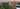The average SAT score of 1465 breaks down into:

• SAT math: 750

The average ACT score for Georgia Institute of Technology is 33 and their acceptance rate is 22.6%.

## Emory University SAT scores

The average SAT score for Emory University is 1435.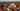The average SAT score of 1435 breaks down into:

• SAT math: 740

The average ACT score for Emory University is 33 and their acceptance rate is 18.5%.

## University of Georgia SAT scores

The average SAT score for University of Georgia is 1325.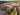The average SAT score of 1325 breaks down into:

• SAT math: 660

The average ACT score for University of Georgia is 30 and their acceptance rate is 48.6%.

## Mercer University SAT scores

The average SAT score for Mercer University is 1263.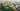The average SAT score of 1263 breaks down into:

• SAT math: 630

The average ACT score for Mercer University is 27 and their acceptance rate is 73.3%.

## Covenant College SAT scores

The average SAT score for Covenant College is 1240.The average SAT score of 1240 breaks down into:

• SAT math: 600

The average ACT score for Covenant College is 27 and their acceptance rate is 97.2%.

## Berry College SAT scores

The average SAT score for Berry College is 1218.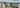The average SAT score of 1218 breaks down into:

• SAT math: 600

The average ACT score for Berry College is 26 and their acceptance rate is 61.6%.

## Oglethorpe University SAT scores

The average SAT score for Oglethorpe University is 1210.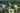The average SAT score of 1210 breaks down into:

• SAT math: 590

The average ACT score for Oglethorpe University is 25 and their acceptance rate is 61.6%.

## Agnes Scott College SAT scores

The average SAT score for Agnes Scott College is 1207.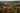The average SAT score of 1207 breaks down into:

• SAT math: 580

The average ACT score for Agnes Scott College is 26 and their acceptance rate is 70.4%.

## Georgia College SAT scores

The average SAT score for Georgia College is 1198.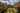The average SAT score of 1198 breaks down into:

• SAT math: 590

The average ACT score for Georgia College is 25 and their acceptance rate is 77.8%.

## Kennesaw State University SAT scores

The average SAT score for Kennesaw State University is 1175.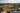The average SAT score of 1175 breaks down into:

• SAT math: 580